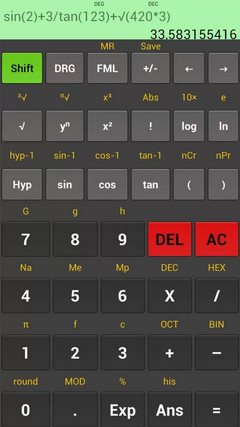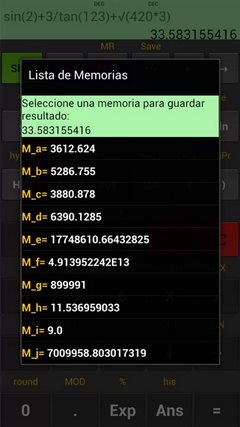# Kal Scientific Calculator APK

Kal Scientific CalculatorScientific calculator with all functions, easy to use, includes a screen to type the characters unlimited transactions, use parentheses and hierarchy in the operations and functions, the result is displayed on the second line of the display. Ability to modify or correct an operation.
Kal Scientific calculator Features:
* Allows function graphs
* New functionality (FML), 100 built-in formulas
* Typical operations (add, subtract, multiply, divide).
* Functions powers (nth power, nth root, squaring, square root, cube root)
* Functions logarithmic (log10, ln, powers of 10, exp)
* Trigonometric functions (sin, cos, tan, including inverse and hyperbolic)
* Three angle modes (DEG, RAD, GRA)
* Random number generator
* PI
* Permutations (nPr) and combinations (nCr)
* Absolute value, factorial.
* Allows numbers in scientific notation.
* Includes major scientific constants.
* Basic operations and converting number systems (decimal, hexadecimal, octal, binary)
* Set decimal number.
* History of the last 10 operations and results.
* Memory storage results

Current Version :  1.33
Size : 577k### 1 Comment

• santosh raj says:

Thanks

This site uses Akismet to reduce spam. Learn how your comment data is processed.